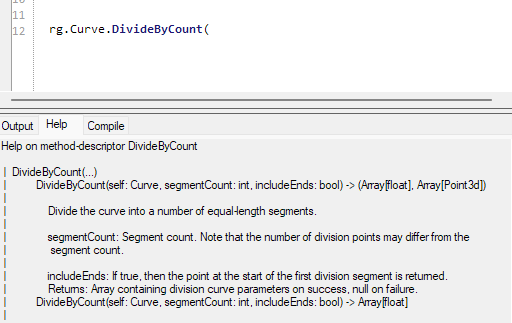# Trying to make a oloid, giving me this problem

Hello everyone, I am new to python in rhino and I am having this problem of the image.

Code:

import Rhino.Geometry as rg
import math

u_values = rg.Interval(0, 2 * math.pi).DivideByCount(100, True)
v_values = rg.Interval(0, 2 * math.pi).DivideByCount(100, True)

points = []

for u in u_values:
for v in v_values:
x = math.cos(u) * math.sin(v)
y = math.sin(u) * math.sin(v)
z = math.cos(v)
point = rg.Point3d(x, y, z)
points.append(point)

mesh = rg.Mesh()

a = mesh


Moved to Grasshopper category.

sorry!

I just wanted to be sure your question was seen by the right people.

2 Likes

If ‘u_values’ and ‘v_values’ are your inputs, why do you immediately ignore and override them?

The error message is crystal clear to me.import Rhino.Geometry as rg
import math

u_values = rg.Interval(0, 2 * math.pi).DivideByCount(100, True)
v_values = rg.Interval(0, 2 * math.pi).DivideByCount(100, True)

points = []

for u in u_values:
for v in v_values:
x = math.cos(u) * math.sin(v)
y = math.sin(u) * math.sin(v)
z = math.cos(v)
point = rg.Point3d(x, y, z)
points.append(point)

mesh = rg.Mesh()
a = mesh


3. Attach minimal versions of all the relevant files

sorry, i don’t know where i override it.

For me it was defining the interval so i get the points and then seting values to position on the formula between the interval.

At the beginning. These two lines ignore and override any input values::

u_values = rg.Interval(0, 2 * math.pi).DivideByCount(100, True)
v_values = rg.Interval(0, 2 * math.pi).DivideByCount(100, True)


i thought it defined the circle region and divide it with 100 points… so you are saying that I should erase this two command lines?

What circle region? Is that hidden in the GH file you didn’t bother to post?

The error message is clear

'interval' object has no attribute 'DivideByCount'

DivideByCount used with curvesGH didn’t have nothing besides the script.

u_values = rg.Interval(0, 2 * math.pi).DivideByCount(100, True)
v_values = rg.Interval(0, 2 * math.pi).DivideByCount(100, True)


i was meaning this part right here.

which command should I use to divide by the count if this one is for curves?

What do you want to do?

a oloid surface

I looked at this image from Wikipedia:

Then tried a few different things and finally settled on creating 1/4 of an Oloid (white group) and mirroring it twice to get a “Closed Brep” (cyan group). I replaced EdgeSrf with NetSrf because it looked better when baked.

oloid_2023Jun3a.gh (23.3 KB)

P.S. Rotated 30 degrees (yellow group) to rest on the XY ground plane:

Some rendering artifacts are still visible using NetSrf2 Likes
1 Like

Hi ，You can generate the list like this.

count = 100
list = rs.frange(0, 2 * math.pi,2 * math.pi/(count-1))

1 Like